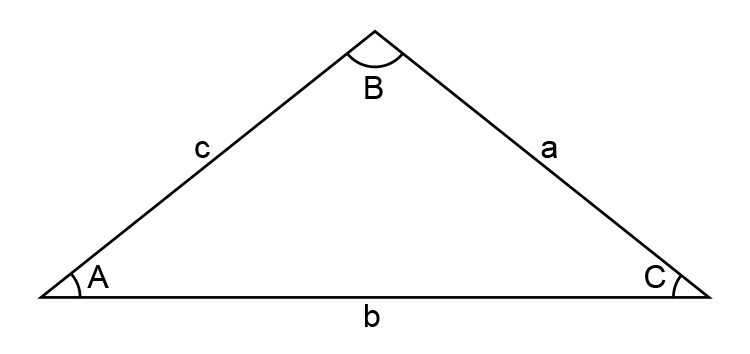# Sine rule

The Sine rule is used to find the length of a side or the size of an angle.a/(sin\ A)=b/(sin\ B)=c/(sin\ C)

The following mnemonic (picture memory aid) is what we recommend to remember this formula.Zero

That’s correct. We recommend nothing. We recommend that you do not remember this formula. You don’t need it.

See examples NOT using Sine rule.